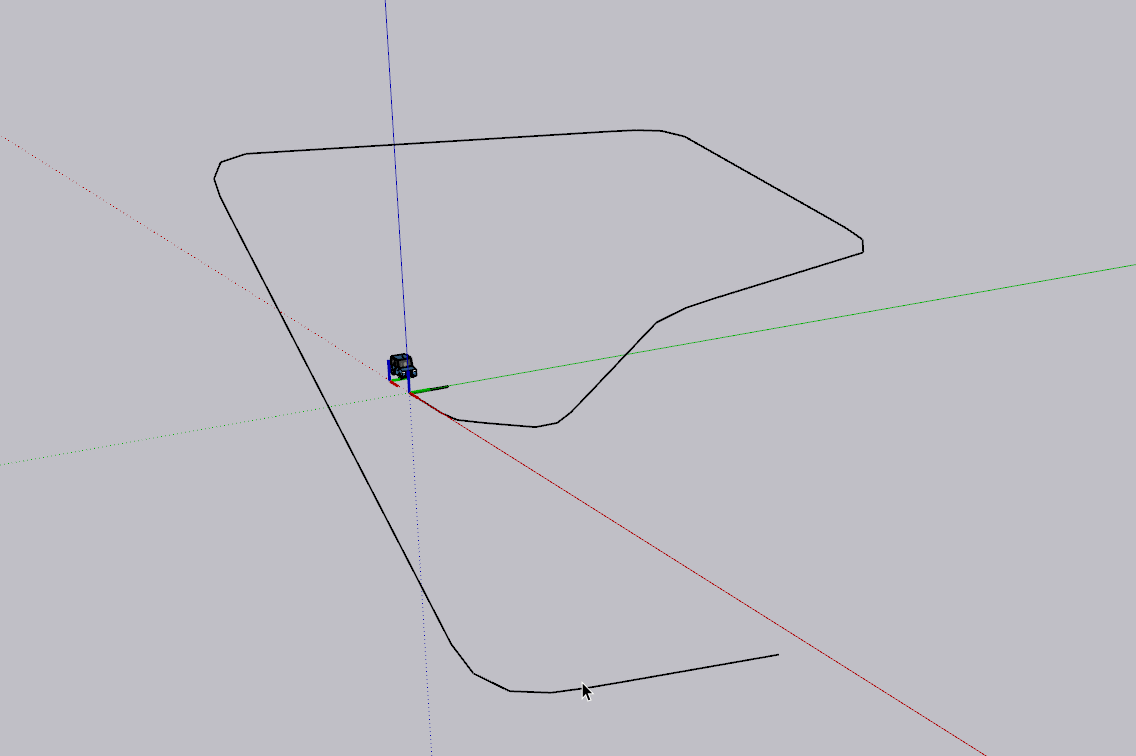# Calculating rotation transformations

Good morning
I need some help calculating rotation transformations.
I have a vehicle that follows a path formed by a non-planar curve.
I want to get the transformation at each point of the curve for the vehicle so that:
Its x axis is collinear with the vector formed by the segment of the curve
Its z axis is collinear with the normal of the plane of the ribbon.

I get there for a plane curve, but not for a 3d curve.
How do you get the angles of rotation

It sounds like you need the angle between any 2 planes. I use the following code to get the acute angle between faces `f1` and `f2` (both `Sketchup::Face` objects):

``````f1_vec, f2_vec = f1.normal, f2.normal
angle = f1_vec.angle_between(f2_vec)
``````

Note that `angle` is expressed in radians. You can convert to degrees by using `angle.radians`.My idea:
Let’s assume you have a

``````# a Sketchup::ComponentInstance :
vehicle

# a Sketchup::Face. :
face

# a Sketchup::Edge (one of the edges that bounds the above face) :
edge

# Geom::Point3d on the above edge (can be e.g.: edge.start or edge.end ):
current_position

``````

Then you can construct a transformation

``````xaxis = edge.line
zaxis = face.normal
yaxis = xaxis * zaxis
origin = current_position
tr = Geom::Transformation.new(xaxis, yaxis, zaxis, origin)
``````

and apply to the `vehicle` instance, considering its current transformation inverse

``````tr_vehicle_inv = vehicle.transformation.inverse
vehicle.transform! tr * tr_vehicle_inv
``````

I’m watching tonight, I’m still on my construction site.

Initially I only have the curve. The planes are those that carry pn pn-2 pn-1 if they are collinear then axisz= ZAXIS

Can you post it? How did you constructed the ribbon from it?
(actually the model of the screenshot above would be better to share)

What is these "pn"s you mention? Are these the curve vertices or edges (curve segments)? I cant interpret this sentence…What is the “axisz”? The z axis of the vehicle?

Hello Dezmo
n means the nth in French mathematics

Here is the code to create the ribbon and place the car on the ribbon for each point on the curve.
There are sometimes errors if the curve undergoes too sudden variations

``````
model = Sketchup.active_model
ents = model.entities

car = model.definitions.filter{|d|d.name == "car"}

#spline is a fredo spline in a group
spline = model.selection
curve= spline.entities.curve

gents = group.entities

# initialisation offset point
p0b = []
p1b = []

for i in 0..curve.edges.length do
p0 = curve.edges[i].start.position
p1 = curve.edges[i].end.position
xaxis= (p0.vector_to(p1)).normalize
origin = p1
if i==0
zaxis = Z_AXIS
else
pm1 = curve.edges[i-1].start.position
vect = pm1.vector_to p0
zaxis = vect*xaxis

if zaxis.%(Z_AXIS) < 0
zaxis.reverse!
end
if (zaxis.angle_between(Z_AXIS)).abs() >= Math::PI/4
zaxis = xaxis*zaxis
end
if zaxis.%(Z_AXIS) < 0
zaxis.reverse!
end
end

yaxis = (xaxis*zaxis).reverse

if i==0
p0b = p0.offset(yaxis,1.m)
else
p0b = p1b
end
p1b = p1.offset(yaxis,1.m)

tr = Geom::Transformation.new(xaxis, yaxis, zaxis, origin)
end
``````

Would be more easy to imagine and analyse, if I get the model (contain the `spline` and `car`), if it is not a top secret project…… Can you share the .skp file?

Hi Dezmo
No nothing “Top secret” it’s learning Ruby!
The idea is to find a generic version of path tracking by a component, to make animation. So whatever the curve, it has to be consistent. I don’t have a precise model, I change the curve each time to see the result.

I see…Learning Ruby is a good pastimeYou can use the segments of the curve as xaxis and use the Vector3d #axes method to compute an arbitrary set of axes with the given vector as the z-axis direction. Then swap these vectors so that the directions are become as needed…

Hi Dezmo
Here is a variant with followme of a face along the curve.
the face must be with a little height !``````model = Sketchup.active_model
ents = model.entities

car = model.definitions.filter{|d|d.name == "car"}
face = model.selection.grep(Sketchup::Face)
#spline is a fredo spline in a group
spline = model.selection.grep(Sketchup::Group)
curve= spline.entities.curve

facepoints = face.vertices.map{|v| v.position}
curvepoints = curve.vertices.map{|v| v.position}

gents = group.entities

faces = gents.grep(Sketchup::Face)

for i in 0..curvepoints.length do
p0 = curvepoints[i]
p1 = curvepoints[i+1]
xaxis= (p0.vector_to(p1)).normalize
origin = p1
face_r = faces.filter{|f| f.vertices.map{|v|v.position}.include?(p0) && f.vertices.map{|v|v.position}.include?(p1)}.sort_by!{|f| f.area}.reverse
zaxis = face_r.normal
yaxis = (xaxis*zaxis).reverse

tr = Geom::Transformation.new(xaxis, yaxis, zaxis, origin)
end
``````
1 Like

Purpose: An animation of a component along a curve

1 Like

Here is a Downhill version of Bobsleigh!
The on-board camera at its axis of vision which follows the rolling movement of the bobsleigh in turns2 Likes